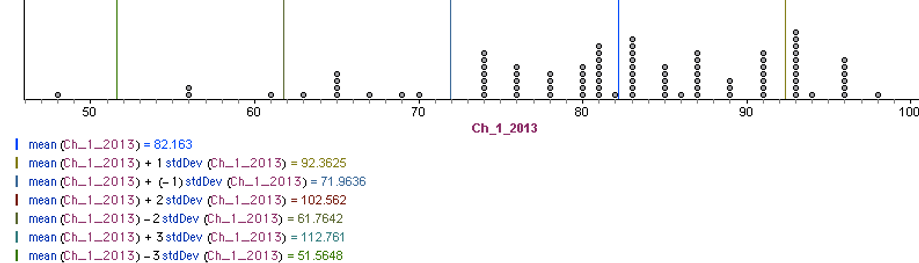## Day 15 - Lesson 2.2

##### Learning Targets
• Determine whether a distribution of data is approximately Normal from graphical and numerical evidence.

Since Normal distribution calculations are so critical to our future success, we decided to do some more practice in class.  Essentially we want to make sure that every student can (1) find area given a value and (2) find a value given an area.  We used this worksheet.  As groups (pairs) finished, we had them put solutions on the board.  And now the grand reveal:

“Students…last night I was searching the Internet using the Google and I found out that there is are calculator functions that will do all this work for us.  Would you like me to show you?”

We used the four problems on the board to show students the calculator functions that could be used to solve each one.

1. normalcdf (1.75, 1.85, 1.84, 0.07)

2. normalcdf (-9999, 1.72, 1.84, 0.07)

3. normalcdf (2, 9999, 1.84, 0.07)

4. invNorm(0.03, 1.84, 0.97)

So when do we want our students to use these calculator functions?

1. Multiple choice if they feel comfortable

2. To check their answer on a free response questions.  For full credit, we want them to show work (see previous lesson for details).

Yes…it is true that you can get full credit on the AP Exam with just the calculator command (if you identify each parameter), but do we want “full credit” or “full understanding”.

##### Activity:

Here is the typical progression for students:

• Type numbers in computer or calculator.

• Make histogram or boxplot.  Check shape.

• Find summary statistics. Compare mean and median.

• Somehow use the 68-95-99.7 rule.

Only the sharpest groups will get to all of these ideas.  Call time at 15 minutes and have groups share out their strategies.  Usually 1 or 2 groups will get to the idea of using the 68-95-99.7 rule.  You can use the One Quantitative Variable applet  to quickly make a dotplot and find summary statistics.  Better yet, use a Fathom file:

First, you will have to add your Chapter 1 Test scores to the top of this document.  Students always love working with data that represents them.  Allow students to work in groups (or pairs) on this Activity.  There is only one question and it is intentionally open ended with no clear correct answer.  Tell students that this Activity is more about their justification than their decision.  The solution with the most support will win (a candy bar).After some discussion, use this as your opportunity to introduce the normal probability plot on the calculator.

##### Teaching Tip

We didn’t spend a lot of time developing how the normal probability plot is constructed (plotting test scores vs. expected z-score).   We really just want students to be able to use this as a tool for assessing normality.  We will need it in Chapters 9, 10 for assessing normality of a sample and in Chapter 12 when we assess the normality of the residuals.

##### Common Student Error

“It is normal because it is linear”

We tell students that “it” is a bad word.  They get a 1 hour detention for inappropriate language.  What we really want here is:

“The distribution of (variable name) is approximately normal, because the normal probability plot is fairly linear”

Notice that we eliminated the inappropriate language and also added -ly words to soften up our claim.